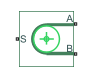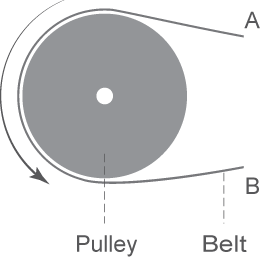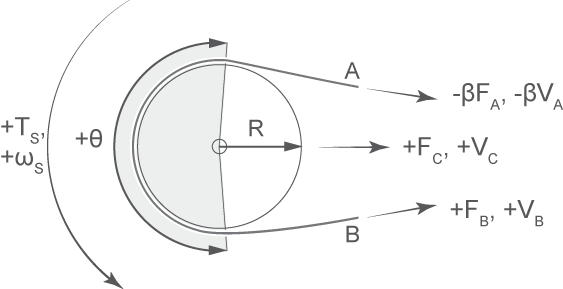# Belt Pulley

Power transmission element with frictional belt wrapped around pulley circumference

•Libraries:
Simscape / Driveline / Couplings & Drives

## Description

The Belt Pulley block represents a pulley wrapped in a flexible ideal, flat, or V-shaped belt. When you set Belt type to `Ideal - No slip`, the belt does not slip relative to the pulley surfaces. You can optionally enable pulley linear translation.

The block accounts for friction between the flexible belt and the pulley surface. The tensioner slips when the load exceeds the friction force. The block accounts for centrifugal loading in the flexible belt, pulley inertia, and bearing friction.The belt ends can either move in the same direction or in the opposite direction.

The block equations relate power transmission between the belt branches or to or from the pulley. The driving and driven branches use the same calculation. Without sufficient tension, the frictional force is not enough to transmit power between the pulley and belt.

Your model is valid when both ends of the belt are in tension. You can choose to display a warning in the Simulink® Diagnostic Viewer when the leading belt end loses tension. When assembling a model, ensure that tension is maintained throughout the simulation by adding mass to at least one of the belt ends or by adding a tensioner like the Cable block. Use the Variable Viewer to ensure that any springs attached to the belt are in tension. For more details on building a tensioner, see Best Practices for Modeling Pulley Networks.

You can set Friction model to `Modal` to use a modal parameterization for the pulley. Choose the modal parameterization for greater numerical robustness. To enable this parameter, set Pulley translation to `Off`.

The Belt Pulley block uses a composite implementation of the Fundamental Friction Clutch block to produce the conditions for the modal parameterization.

### Equations

For either setting of the Friction model parameter, the block equations refer to these quantities:

• β is the belt direction sign. When you set Belt direction to ```Ends move in same direction```, β = 1. Otherwise, β = -1.

• Vrel is the relative velocity between the belt and pulley periphery. Vrel = 0 when Belt type is ```Ideal - No slip```.

• VA is the linear velocity of branch A.

• VB is the linear velocity of branch B.

• VC is the linear velocity of the pulley at its center. If Pulley translation is `Off`, the block constrains this to 0.

• ωS is the angular velocity of the pulley contact surface.

• R is the radius of the pulley.

• Fcentrifugal is centrifugal force of the belt.

• FC is the force acting through the pulley centroid. When you specify a value for Inertia, FC includes force due to the pulley mass acceleration.

• ρ is the belt linear density.

• Ffr is the friction force between the pulley and the belt.

• FA is the force acting along branch A.

• FB is the force acting along branch B.

• θ is the contact wrap angle.

• τS is the pulley torque.

The kinematic constraints between the pulley and belt are:

`$-\beta {V}_{A}={V}_{C}-R{\omega }_{S}-\beta {V}_{rel}$`

`${V}_{B}={V}_{C}+R{\omega }_{S}+\beta {V}_{rel}$`

When you set Belt type to either `V-belt` or `Flat belt` and set Centrifugal force to `Model centrifugal force`, the centrifugal force is:

`${F}_{centrifugal}=\rho {\left({V}_{B}-{V}_{C}\right)}^{2}.$`

When you set Pulley translation to `On`, the force balancing equation is:

`${F}_{C}=\left(\beta {F}_{A}-{F}_{B}-{F}_{centrifugal,smooth,sat}\right)\cdot \mathrm{sin}\left(\frac{\theta }{2}\right).$`

To calculate Fcentrifugal,smooth,sat, the block

• Smooths Fcentrifugal using Fthr. You can increase smoothing by raising Fthr, and you can decrease smoothing by lowering Fthr.

• Saturates values above βFA-FB.The sign convention is such that, when Belt direction is `Ends move in opposite direction`, a positive rotation in port S results in a negative translation for port A and a positive translation for port B.

To enable the Friction model parameter, set Belt type to `Flat belt` or `V-belt` and Pulley translation to `Off`.

Continuous Friction

When you set Friction model to `Continuous`, the block equations refer to these quantities:

• μ is the Contact friction coefficient parameter.

• μsmoothed is the instantaneous value of the friction coefficient.

• Vthr is the Velocity threshold parameter.

• b is the viscous damping of the bearing.

• Fthr is the Force threshold parameter.

The instantaneous friction coefficient is a function of the relative velocity such that

`${\mu }_{smoothed}={\mu }_{sheave}\mathrm{tanh}\left(4\frac{{V}_{rel}}{{V}_{thr}}\right),$`

where the hyperbolic tangent function maintains numerical robustness by ensuring a smooth and continuous output for Vrel zero-crossings.

For a V-belt, the block derives the contact friction value using the sheave angle:

`${\mu }_{sheave}=\frac{\mu }{\mathrm{sin}\left(\frac{\varphi }{2}\right)},$`

where:

• μsheave is the effective friction coefficient.

• Φ is the sheave angle.

For a flat belt, μsheave = μ.

The friction velocity threshold controls the width of the region within which the friction coefficient changes its value from zero to a steady-state maximum. The friction velocity threshold specifies the velocity at which the hyperbolic tangent equals `0.999`. The smaller the value, the steeper the change of μ.

The block determines the effect of friction on the force at the belt ends as:

`$-\beta {F}_{A}-{F}_{centrifugal}=\left({F}_{B}-{F}_{centrifugal}\right){e}^{-{\mu }_{smoothed}\theta },$`

which follows the form of the capstan equation, also known as the Euler-Eytelwein equation. The torque acting on the pulley is

`${\tau }_{S}=\left(-\beta {F}_{A}-{F}_{B}\right)R\sigma +{\omega }_{S}b,$`

where σ = 1 when you set Belt type to `Ideal - No slip`. Otherwise,

`$\sigma =\mathrm{tanh}\left(4\frac{{V}_{rel}}{{V}_{thr}}\right)\mathrm{tanh}\left(\frac{{F}_{B}}{{F}_{thr}}\right).$`

Modal Friction

For a V-belt, the block derives the static and kinetic friction values by using the sheave angle:

`$\begin{array}{l}{\mu }_{Static,sheave}=\frac{{\mu }_{Static}}{\mathrm{sin}\left(\frac{\varphi }{2}\right)}\\ {\mu }_{Kinetic,sheave}=\frac{{\mu }_{Kinetic}}{\mathrm{sin}\left(\frac{\varphi }{2}\right)}\end{array}$`

where:

• μStatic is the Static friction coefficient parameter.

• μStatic,sheave is effective static friction coefficient.

• μKinetic is the Kinetic friction coefficient parameter.

• μKinetic,sheave is effective kinetic friction coefficient.

• Φ is the sheave angle.

For a flat belt, μStatic,sheave = μStatic, and μKinetic,sheave = μKinetic.

When you set Friction model to `Modal`, the block calculates the maximum static friction force before slipping as

`${F}_{Friction,Static,Max}=\left({F}_{Drive}-{F}_{Centrifugal}\right)\cdot \left(1-{e}^{-{\mu }_{Static,sheave}\theta }\right),$`

where FFriction,Static,Max is the maximum force magnitude due to static friction, and FDrive is the force due to tension felt at the end of the pulley with the greatest force magnitude. As with Fcentrifugal, the block uses Fthr to smooth FDrive. To increase or decrease the smoothing on FDrive, raise of lower the value of the Force threshold parameter.

The block smoothly saturates FFriction,Static,Max to be greater than or equal to 0 as

`${F}_{Friction,Static,Max,Smooth}=0.5\left({F}_{Friction,Static,Max}+\sqrt{{F}_{Friction,Static,Max}^{2}+{\left(R{F}_{thr}\right)}^{2}}\right).$`

During slip,

`${F}_{Friction,Slip}=\frac{{\mu }_{Kinetic,sheave}}{{\mu }_{Static,sheave}}{F}_{Friction,Static,Max},$`

where μKinetic,sheave is the kinetic friction coefficient. The block resolves the torque balance using

`${\tau }_{S}={\omega }_{S}b+R{F}_{Friction},$`

and

`$R\beta {F}_{A}+R{F}_{B}=-R{F}_{Friction},$`

where Simscape™ logs RFFriction as `fundamental_clutch.torque`.

## Assumptions and Limitations

• The block assumes noncompliance along the length of the belt.

• The block assumes both belt ends maintain adequate tension throughout the simulation.

• The block treats the translation of the pulley center as planar where the pulley travels along the bisect of the pulley wrap angle. The center velocity VC and force FC only account for the component along this line of motion.

• The Eytelwein equation for belt friction neglects the effect of pulley translation on friction.

## Ports

### Conserving

expand all

Mechanical rotational conserving port associated with the angular velocity of the pulley shaft.

Mechanical translational conserving port associated with the linear velocity of belt end A.

Mechanical translational conserving port associated with the linear velocity of belt end B.

Mechanical translational conserving port associated with pulley translation. The pulley moves in the plane and along the bisect of the pulley wrap angle. When the relative velocity is positive, the pulley center moves.

#### Dependencies

To enable this port, set Pulley translation to `On`.

## Parameters

expand all

### Belt

Belt type selection. The belt type affects slip conditions.

• `Ideal - No slip` — Parameterize an ideal belt that does not slip relative to the pulley.

• `Flat belt` — Parameterize a belt with a rectangular cross-section.

• `V-belt`— Parameterize a belt with a V-shaped cross-section.

Sheave angle of the V-belt.

#### Dependencies

To enable this parameter, set Belt type to `V-belt`.

Number of V-belts.

The block rounds noninteger values to the nearest integer. Increasing the number of belts increases the effective mass per unit length and maximum allowable tension.

#### Dependencies

To enable this parameter, set Belt type to `V-belt`.

Option to include the effects of centrifugal force. If you set this parameter to `Model centrifugal force`, centrifugal force saturates to approximately 90% of the value of the force on each belt end.

#### Dependencies

To enable this parameter, set Belt type to `Flat belt` or `V-belt`.

Centrifugal force contribution in terms of linear density expressed as mass per unit length.

#### Dependencies

To enable this parameter, set Belt type to ```Flat belt``` or `V-belt` and Centrifugal force to ```Model centrifugal force```.

Relative direction of translational motion of one belt end with respect to the other.

Option to specify a maximum tension. If you select ```Specify maximum tension``` and the belt tension on either end of the belt meets or exceeds the value that you specify for the Belt maximum tension parameter, the simulation stops and generates an assertion error.

Maximum allowable tension for each belt. When the tension on either end of the belt meets or exceeds this value, the simulation stops and generates an assertion error.

#### Dependencies

To enable this parameter, set Maximum tension to `Specify maximum tension`.

Whether the block generates a warning when the tension at either end of the belt falls below zero.

Note

When combining Belt Pulley blocks with Rope blocks to form loops, set the Belt Pulley block Tension warning parameter to `Do not check tension` and set the Rope block Tension warning parameter to ```Warn if rope loses tension```.

### Pulley

Option to allow the pulley to translate. Setting this parameter to `On` enables port C.

Viscous friction associated with the bearings that hold the axis of the pulley.

Option to parameterize the rotational inertia with an initial velocity.

Rotational inertia of the pulley.

#### Dependencies

To enable this parameter, set Inertia to ```Specify inertia and initial velocity```.

Initial rotational velocity of the pulley.

#### Dependencies

To enable this parameter, set Inertia to ```Specify inertia and initial velocity```.

Pulley mass for the inertia calculation.

#### Dependencies

To enable this parameter, set

• Pulley translation to `On`

• Inertia to ```Specify inertia and initial velocity```

Initial translational velocity of the pulley.

#### Dependencies

To enable this parameter, set

• Pulley translation to `On`

• Inertia to ```Specify inertia and initial velocity```

### Contact

To enable these settings, set Belt type to ```Flat belt``` or `V-belt`.

Option to parameterize continuous or modal friction.

#### Dependencies

To enable this parameter, set Pulley translation to `Off`.

Option to initialize the simulation with the pulley locked or unlocked.

#### Dependencies

To enable this parameter, set

• Pulley translation to `Off`

• Friction model to `Modal`

Belt friction while maintaining static contact.

#### Dependencies

To enable this parameter, set Friction model to `Modal`.

Belt friction while slipping.

#### Dependencies

To enable this parameter, set Friction model to `Modal`.

Coulomb friction coefficient between the belt and the pulley surface.

#### Dependencies

To enable this parameter, set Friction model to `Continuous`.

Radial contact angle between the belt and the pulley.

Relative velocity required for peak kinetic friction in the contact. The friction velocity threshold improves the numerical stability of the simulation by ensuring that the force is continuous when the direction of the velocity changes.

Relative force required for peak kinetic friction in the contact.

#### Dependencies

To enable this parameter, set Belt type to `Flat belt` or `V-belt`.